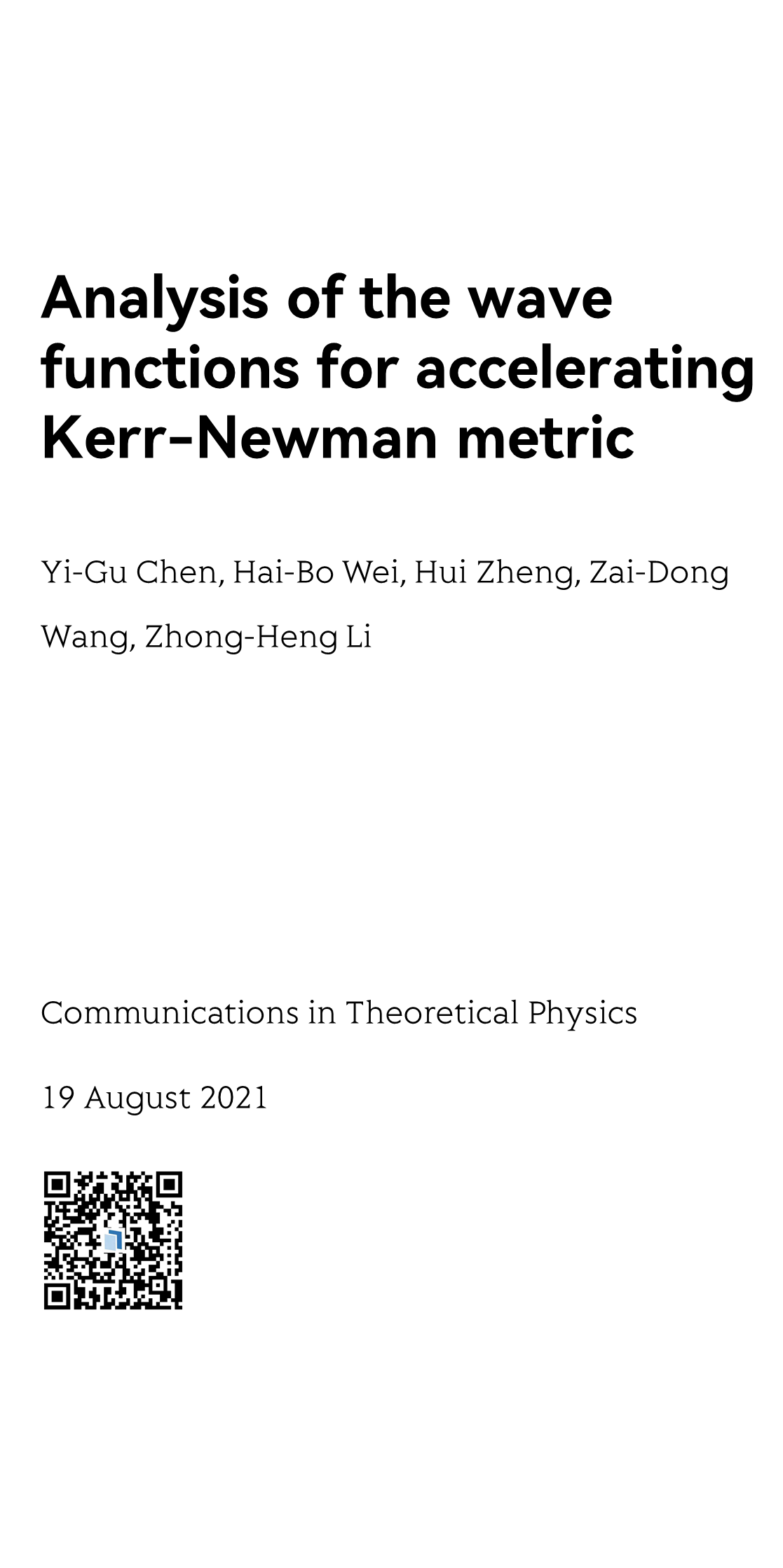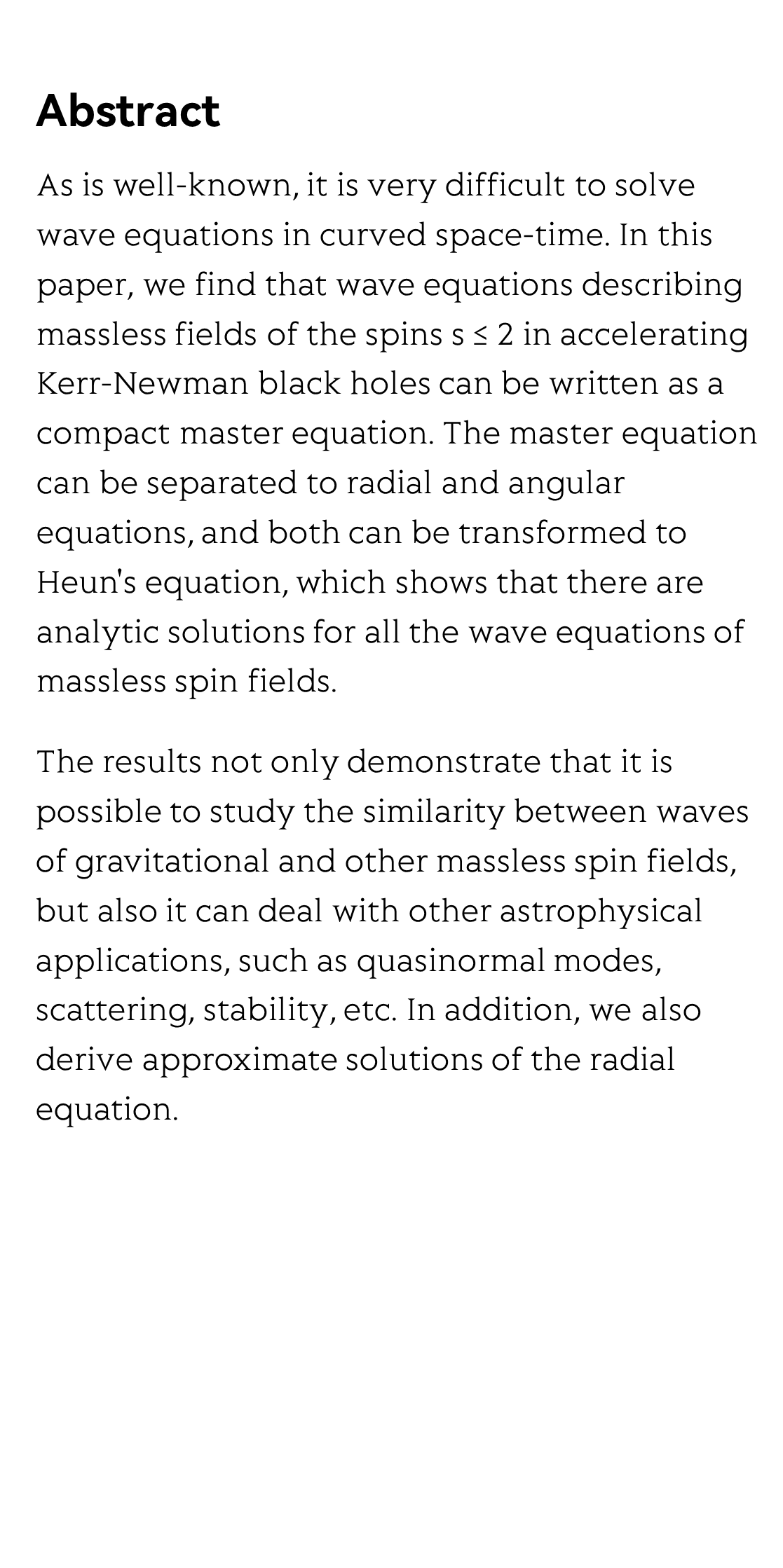MMMYYYYAnalysis of the wave functions for accelerating Kerr-Newman metric

カー・ニューマン解法を加速するための波動関数の分析
Kerr-Newman 메트릭 가속을 위한 파동 함수 분석
Análisis de las funciones de onda para acelerar la métrica de Kerr-Newman
Analyse des fonctions d'onde pour accélérer la métrique Kerr-Newman
Анализ волновых функций для ускоряющей метрики Керра-Ньюмана
Yi-Gu Chen, Hai-Bo Wei, Hui Zheng, Zai-Dong Wang, Zhong-Heng Li 黎忠恒
College of Science, Zhejiang University of Technology, Hangzhou 310023, China

Communications in Theoretical Physics, 19 August 2021Abstract

As is well-known, it is very difficult to solve wave equations in curved space-time. In this paper, we find that wave equations describing massless fields of the spins s ≤ 2 in accelerating Kerr-Newman black holes can be written as a compact master equation. The master equation can be separated to radial and angular equations, and both can be transformed to Heun's equation, which shows that there are analytic solutions for all the wave equations of massless spin fields.

The results not only demonstrate that it is possible to study the similarity between waves of gravitational and other massless spin fields, but also it can deal with other astrophysical applications, such as quasinormal modes, scattering, stability, etc. In addition, we also derive approximate solutions of the radial equation.Reviews and Discussions